#### Angular velocity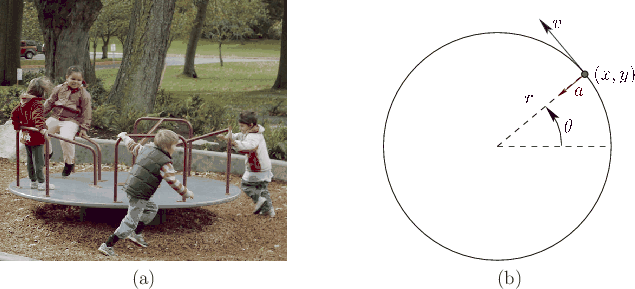To understand the issues, consider the simple case of a spinning merry-go-round, as shown in Figure 8.2(a). Its orientation at every time can be described by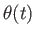; see Figure 8.2(b). Let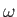denote its angular velocity: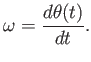(8.10)

By default,has units of radians per second. If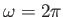, then the rigid body returns to the same orientation after one second.

Assuming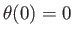andis constant, the orientation at time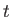is given by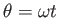. To describe the motion of a point on the body, it will be convenient to use polar coordinates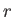and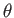: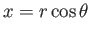and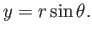(8.11)

Substitutingyields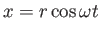and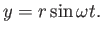(8.12)

Taking the derivative with respect to time yields8.2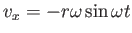and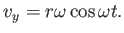(8.13)

The velocity is a 2D vector that when placed at the point is tangent to the circle that contains the point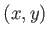; see Figure 8.2(b).

This makes intuitive sense because the point is heading in that direction; however, the direction quickly changes because it must move along a circle. This change in velocity implies that a nonzero acceleration occurs. The acceleration of the pointis obtained by taking the derivative again: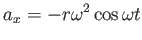and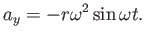(8.14)

The result is a 2D acceleration vector that is pointing toward the center (Figure 8.2(b)), which is the rotation axis. This is called centripetal acceleration. If you were standing at that point, then you would feel a pull in the opposite direction, as if nature were attempting to fling you away from the center. This is precisely how artificial gravity can be achieved in a rotating space station.

Steven M LaValle 2020-11-11